Before getting started, suppose you are blindfolded and asked to pick up a pen from a table. The only clue you are given is that the pen is at a distance of one arm from you. Would you be able to pick it up in one go? Absolutely not! Of course, you “may” pick it up but it’s uncertain. You may end up swinging your arm in one arm’s radius of yours.

So now, would you be able to pick up the pen having said that it’s at one arm’s distance in front of you?  Seems possible right? This is exactly where the application of vectors in our real-life comes into the picture unknowingly. Wondering how? Let me explain.

In the first case when you were told that the pen is at one arm’s distance from you, you only know the magnitude of the distance which is why you end up puzzled in locating the pen. But in the second case, along with the magnitude of the distance, you know the direction(in front of you) as well which makes your task a cakewalk.

Vectors and scalars are the two main types of quantities. There is a difference between vectors and scalars: vectors have both direction and magnitude whereas scalars only have magnitude. A vector can be visualized as a directed line segment whose length equals the magnitude of the vector, with an arrow indicating its direction. The direction of the vector is from its tail to its head.Two vectors are the same if they have the same magnitude and direction. This means that if we take a vector and translate it to a new position (without rotating it), then the vector we obtain at the end of this process is the same vector we had in the beginning. Thus, It is essential to know what kind of and how many different operations can be performed on these quantities. Let’s look at some of these operations in detail.

We can represent vectors graphically as arrows and then the sum of two vectors is found (graphically) by joining the head of one to the tail of the other and then connecting head to tail for the combination.

Let us see that how vectors look likehorizontally and vertically it looks likeNow let’s see how to define a vector in S2.

				
%use s2
// define a vector
var a = DenseVector(arrayOf(1.0, 3.0))
var b = DenseVector(arrayOf(2.0, 1.0))

println(a)
println(b)


OUTPUT: [1.000000, 3.000000] [2.000000, 1.000000]

## Vector Norm

The vector norm or magnitude is the length of the vector. Vector length is a nonnegative number that describes the extent of the vector in space, and it is sometimes called the magnitude or norm of the vector.

The formula to find norm of a vector is as follows The norm of a vector is simply the square root of the sum of each component squared. Below are some properties of norm vector.

Vectors always have a positive norm or zero ‖ a ‖ ⩾ 0 . A vector has a zero norm if and only if it is a zero vector. Scalar multiples of a norm are equal to the product of the absolute value of the scalar and the norm ‖ k a ‖ = | k | ‖ a ‖ .

				
%use s2
//define a vector to find its norm
var v = DenseVector(arrayOf(3.0, 4.0))
//get the components of the vector
val x = v.get(1)
val y = v.get(2)
//calculate the square root of the sum of the squares of vector's components
val norm = (x.pow(2.0) + y.pow(2.0)).pow(0.5)
print(norm)


Output: 5.0

2. Vector Subtraction
3. Scalar Multiplication

The usual algebraic rules cannot be used to add vectors. A vector’s magnitude and direction must be considered when adding two vectors. The diagram below shows two vectors “a” and “b” and the resultant calculated “c” after they are added. In vector addition, the resultant vector is independent of the order in which the two vectors are added. Lets see the vector addition operation numerically.

Given the formula for addition of two vectors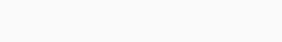Then the sum is,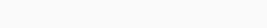Then if vectors areBy using the formula let’s Add those VectorsLet’s plot the above equation on graph.

After adding those vectors we will see something like this which exactly replicates our numerical solution.When we follow the rule to attach the tail of one vector to the head of another vector we will see the resulting vector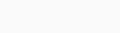on b after addition where b has the same magnitude and direction.

				
%use s2
// define 2 vectors
var v1 = DenseVector(arrayOf(1.0, 3.0))
var v2 = DenseVector(arrayOf(2.0, 1.0))
println(vec_sum)


Output: [3.000000, 4.000000]

## Vector Subtraction

We will follow the same procedure from addition we will first prove by equation, graph, code for better understanding.

Given the formula for Subtraction of two vectors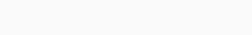Then the difference is,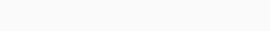Let’s say the vectors areBy using the formula let’s subtract those VectorsNow lets plot those vectors and show the resultant vector using graph

After Subtracting those vectors we will see something like this which exactly replicates our numerical solution.In the subtraction the magnitude is same but the direction is opposite which is reflected in the diagram above. Also you can see, we have represented the same solution as numericals.

Which is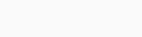Now let’s code it to demonstrate the same using S2

				
%use s2
// define 2 vectors
var v1 = DenseVector(arrayOf(1.0, 3.0))
var v2 = DenseVector(arrayOf(2.0, 1.0))
//subtracting two vectors
val vec_sub=v1.minus(v2)
println(vec_sub)


Output: [-1.000000, 2.000000]

## Scalar Multiplication

In mathematics, scalar multiplication is one of the basic operations defining a vector space in linear algebra. It multiplies the magnitude of the vector without changing its direction. The term “scalar” itself derives from this usage: a scalar is that which scales vectors. Scalar multiplication is the multiplication of a vector by a scalar (where the product is a vector), and is to be distinguished from the inner product of two vectors (where the product is a scalar).

The formula is as follows: To multiply a vector by a scalar, multiply each component by the scalar. If →u=⟨u1,u2⟩ has a magnitude |→u| and direction d , then n→u=n⟨u1,u2⟩=⟨nu1,nu2⟩ where n is a positive real number, the magnitude is |n→u| , and its direction is d .

If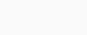then scalar multiplication will be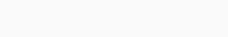Let’s see the above equation in the form of graphsThe graph shows the exact solution of Scalar multiplication as of equation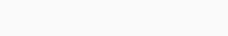%use s2
// define a vector
var v = DenseVector(arrayOf(1.0, 2.0))
//define a scalar
val s = 2.0
// B = v*s
val B = v.scaled(s)
println(B)


Output: [2.000000, 4.000000]

Note:

1. Multiplying a vector by a positive real number k preserves its direction and multiplies its norm by k.
2. Multiplying a vector by a negative real reverses its direction and multiplies its norm by k.
3. Multiplying a vector by -1 reverses its direction and preserves its norm.

Let us illustrate an example implementing the operations involved in the concepts explained above.

Ever played games like Catapult Quest or Angry Birds? If you keenly observe, in games, vectors are used to store positions, directions and velocities just like the one below.

• The position vector indicates how far the object is.
• The velocity vector indicates the time taken or the amount of force that must be given.
• The direction vector indicates the direction in which the force should be applied.

Linear Combination

Let us define a list of vectors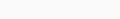. The linear combination of these vectors is expressed as: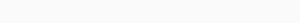whereare real numbers.

These $$c$$’s are called the weights of the linear combination.

				
%use s2
// define vector v1
var v1 = DenseVector(arrayOf(1.0, 2.0))
//define c1
val c1 = 3.0
//Repeat the same for required number of terms
var v2 = DenseVector(arrayOf(3.0, 3.0))
val c2 = 1.0
var v3 = DenseVector(arrayOf(5.0, 3.0))
val c3 = 2.0
// Scalar Multiplication
val A = v1.scaled(c1)
val B = v2.scaled(c2)
val C = v3.scaled(c3)
println(sol)


Output: [16.000000, 15.00000]

Let us illustrate the same using graph for a better understanding.Fig 4: Linear Combination of vectors

Note: An integer linear combination is a linear combination in which all the weights (c’s) are integers.

Span

The span of a given list of vectors is defined as the set of all vectors which can be written as the linear combination of the given list of vectors.

Ex: $$\begin{bmatrix}x\\y\end{bmatrix}$$ is said to be in the span of the vector-list $$\left\{\begin{bmatrix}x_1\\y_1\end{bmatrix},\begin{bmatrix}x_2\\y_2\end{bmatrix}, \begin{bmatrix}x_3\\y_3\end{bmatrix}\right\}$$ if:

$$\begin{bmatrix}x\\y\end{bmatrix} = \alpha \begin{bmatrix}x_1\\y_1\end{bmatrix}+\beta \begin{bmatrix}x_2\\y_2\end{bmatrix}+\gamma \begin{bmatrix}x_3\\y_3\end{bmatrix}$$

where $$\alpha, \beta, \gamma$$ ∈ $$\mathbb{R}$$.

Let us solve a problem to improve our understanding.

Q: Determine whether the vector $$\begin{bmatrix}19\\10\\-1\end{bmatrix}$$ lies in the span of set of vectors: $$S = \left\{\begin{bmatrix}3\\-1\\2\end{bmatrix}, \begin{bmatrix}-5\\0\\1\end{bmatrix}, \begin{bmatrix}1\\7\\-4\end{bmatrix}\right\}$$.

Approach:

Let us first assume the equation: $$c_1v_1+c_2v_2+c_3v_3 = v$$, where $$c_1, c_2, c_3$$ are real numbers and try to find the solution $$[c_1, c_2, c_3]$$ if exists.

				
%use s2
// define vectors v1,v2,v3,v
var v1 = DenseVector(arrayOf(3.0, -1.0, 2.0))
var v2 = DenseVector(arrayOf(-5.0, 0.0, 1.0))
var v3 = DenseVector(arrayOf(1.0, 7.0, -4.0))
var v = DenseVector(arrayOf(19.0, 10.0, -1.0))
//we need to determine whether there exists a solution for the equation: c1v1+c2v2+c3v3 = v
//for real numbers c1,c2,c3.
// Create a 3x3 matrix for v1,v2,v3
val A = DenseMatrix(arrayOf
(doubleArrayOf(3.0, -5.0, 1.0),
doubleArrayOf(-1.0, 0.0, 7.0),
doubleArrayOf(2.0, 1.0, -4.0)))
//now we need to find whether there exists a solution for Ac=v where c = {c1, c2, c3}
//and if yes, print [c1, c2, c3]
// Create a solver for linear system
val precision = 1e-15
val solver = LinearSystemSolver(precision)
// Solve for Ac
val soln = solver.solve(A)
// solution for Ac = v
val c = soln.getParticularSolution(v)
println("soln is: $c") // verification val Ac = A.multiply(c) // Ac = v println("Ac =$Ac, same as \$v")



soln is: [4.000000, -1.000000, 2.000000]

Ac = [19.000000, 10.000000, -1.000000] , same as [19.000000, 10.000000, -1.000000]